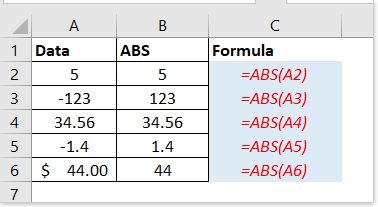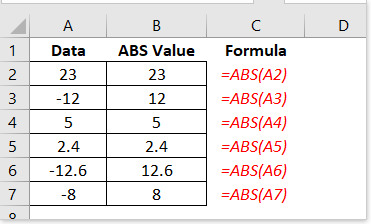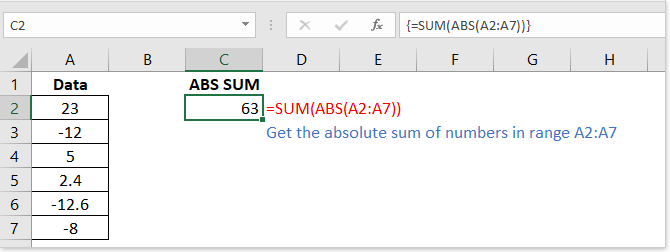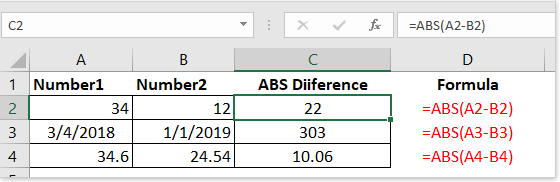## Excel ABS functionDescription Syntax and Arguments Usage and Examples

### Description

The ABS function returns the absolute value of a number. Negative numbers will be converted to positive with this function, but positive numbers and zero number will be unaffected.

### Syntax and arguments

Formula syntax

 =ABS(number)

Arguments

 Number: Required. The number whose absolute value you want to get.

Return Value

The ABS function returns the absolute value of the given number.

### Usage and Examples

In this section, I provide some practical applications for using the ABS function in Excel.

Example 1: Get the absolute value of numbers (convert negative numbers to positive numbers)

Formula

=ABS(A2)

Explain: Convert the number in A2 to absolute value (positive value).

You can drag the fill handle down to the cells which you want to apply this formula.Example 2: Get sun/average of absolute value in Excel

Get the absolute sum of numbers in a range.

Array formula syntax:

=SUM(ABS(range))

Press Shift + Ctrl + Enter keys at the same time to get the correct calculation

Take getting absolute sum of numbers in A2:A7 as instance, enter formula

=SUM(ABS(A2:A7))

And press Shift + Ctrl + Enter keys simultaneously to get the correct result.Tip:

1. you also can use this formula =SUMPRODUCT(ABS(A2:A7)) and press the Enter key to get absolute sum.

2. If you want to get absolute average value of numbers in a range, use this array formula
=AVERAGE(ABS(range)),
and press Shift + Ctrl + Enter keys simultaneously.

Example 3: Get absolute difference between numbers/dates in Excel

Formula Syntax

=ABS(number1-number2)

Arguments

Number1, number2: Required. The two numbers whose absolute difference you want to get.

Take below screenshot for instance：

Calculate the absolute difference of A2 and B2, use this formula

=ABS(A2-B2)Tip: if you want to calculate the absolute difference between two times, use =ABS(number1-number2), and format the results to time.

### The Best Office Productivity Tools

#### Kutools for Excel - Helps You To Stand Out From Crowd

 Popular Features: Find, Highlight or Identify Duplicates  |  Delete Blank Rows  |  Combine Columns or Cells without Losing Data  |  Round without Formula ... Super VLookup: Multiple Criteria  |  Multiple Value  |  Across Multi-Sheets  |  Fuzzy Lookup... Adv. Drop-down List: Easy Drop Down List  |  Dependent Drop Down List  |  Multi-select Drop Down List... Column Manager: Add a Specific Number of Columns  |  Move Columns  |  Toggle Visibility Status of Hidden Columns  |  Compare Columns to Select Same & Different Cells ... Featured Features: Grid Focus  |  Design View  |  Big Formula Bar  |  Workbook & Sheet Manager | Resource Library (Auto Text)  |  Date Picker  |  Combine Worksheets  |  Encrypt/Decrypt Cells  |  Send Emails by List  |  Super Filter  |  Special Filter (filter bold/italic/strikethrough...) ... Top 15 Toolsets:  12 Text Tools (Add Text, Remove Characters ...)  |  50+ Chart Types (Gantt Chart ...)  |  40+ Practical Formulas (Calculate age based on birthday ...)  |  19 Insertion Tools (Insert QR Code, Insert Picture from Path ...)  |  12 Conversion Tools (Numbers to Words, Currency Conversion ...)  |  7 Merge & Split Tools (Advanced Combine Rows, Split Excel Cells ...)  |  ... and more

Kutools for Excel Boasts Over 300 Features, Ensuring That What You Need is Just A Click Away...#### Office Tab - Enable Tabbed Reading and Editing in Microsoft Office (include Excel)

• One second to switch between dozens of open documents!
• Reduce hundreds of mouse clicks for you every day, say goodbye to mouse hand.
• Increases your productivity by 50% when viewing and editing multiple documents.
• Brings Efficient Tabs to Office (include Excel), Just Like Chrome, Edge and Firefox.